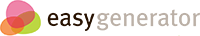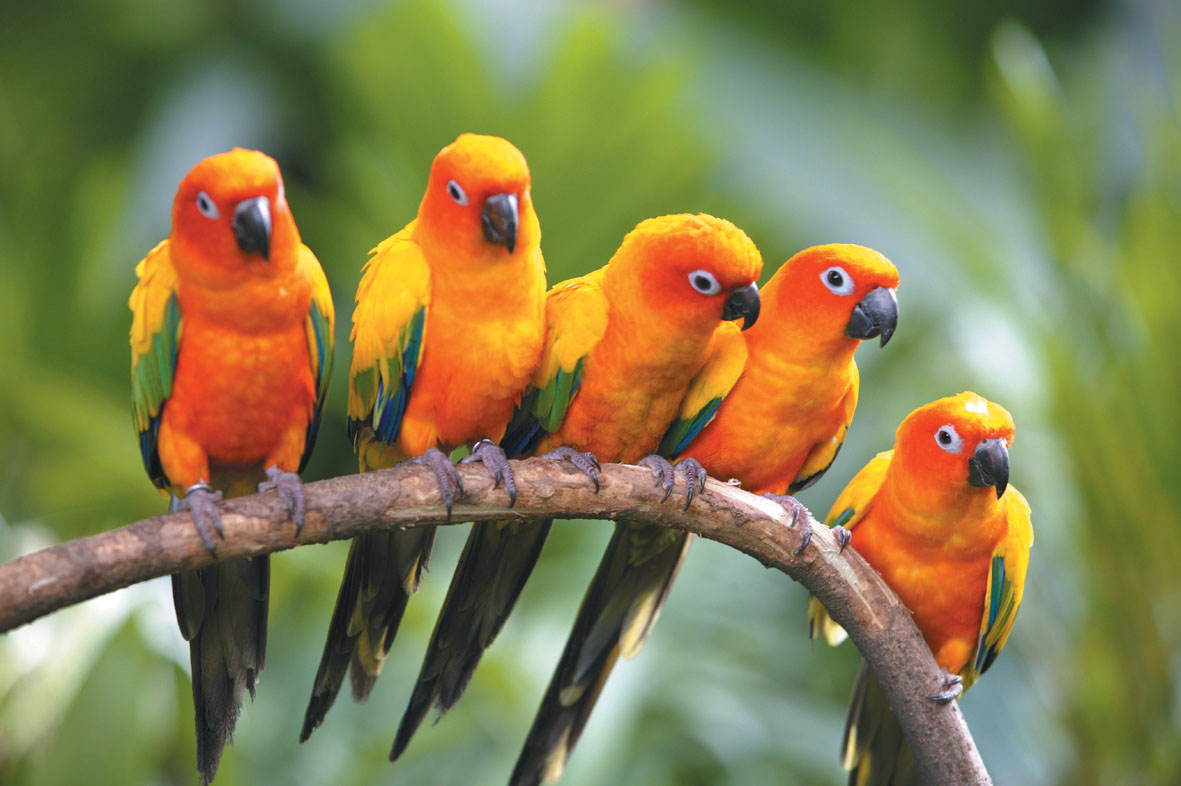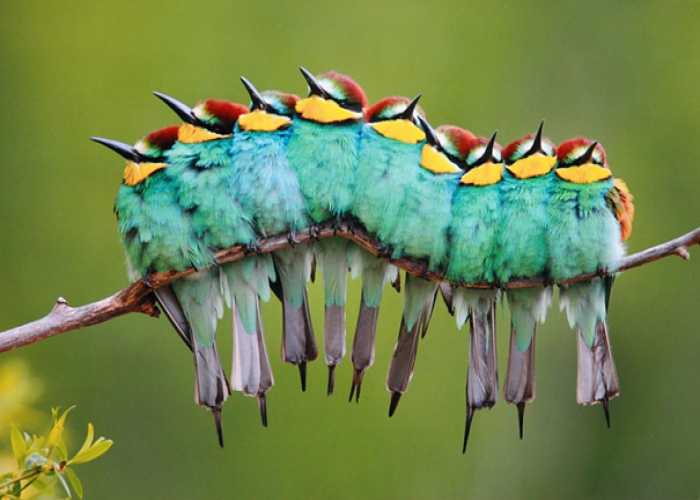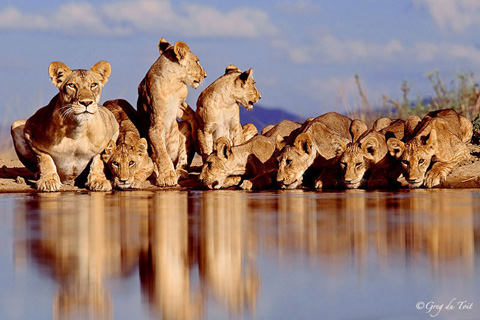# Focus on Picture Addition and Addition Combinations till 18

## Introduction to Addition using Concrete Objects and Pictures

### Decompose and Click the correct choice

• 3 + 1 = 4
• 2 + 3 = 5
• 1 + 4 =5
• 3 + 2 = 5Click the Addition Fact which does not represent the above picture fact? Decompose and answer

### Decompose the Flying Beauties

• 4 + 5 =9
• 3 + 5 =9
• 6 + 2 = 9
• 7 + 3 =9Let us look at the picture which has some flying beauties together. Now you group these flying beauties to two groups and get a addition fact. Make all such decomposition and answer which is correct statement?

### Decompose and Click all the correct Addition Facts

• 6+0=6
• 3+3=6
• 4+2=6
• 1+5=6
• 2+3=6### Decompose into more than three groups

• 3 + 4 +5=12
• 5 + 5 + 2=12
• 4 + 4 + 4 =12
• 4 + 6 + 2 = 12This is a group of Siberian Tigers. Now you need to group them into three groups and click on the options which are correct ( This is a difficult activity. Student can also use Number Chips along with this activity )

## Strategies for Making Number Bonds of 3,4,5 and Picture Subtraction

### Decompose and Click the correct choice

• 3 + 4=8
• 3 + 5 = 8
• 4 + 4 =8
• 2 + 6 =8
• 1 + 7 =8Decompose into two groups and make an addition facts. From those click which of them are not correct

### Addition Facts from Picture Facts

• 5 + 4 =8
• 4 + 5 =10
• 5 + 4 =8
• 5 + 4 =9Click  the correct representation of addition facts?

### Addition Facts from Picture Facts

• 6 + 8 =13
• 6 + 8 =14
• 8 + 7 = 14
• 8 + 7 = 13Click  the correct representation of addition facts?

### Addition Facts from Picture Facts

• 9 + 8 = 17
• 8 + 9 = 16
• 8 + 9 = 15
• 9 + 8 = 15Click  the correct representation of addition facts?

### Subtraction Facts from Picture Facts

• 6 - 5 =1
• 5 -6 =1
• 6 -2 =4
• 6 -3 =1The correct subtraction fact for the above picture fact is

### Subtraction Facts from Picture Facts

• 4 -4 =0
• 4 - 3 =1
• 3 - 3 =0
• 3 - 2 = 1The correct subtraction fact for the above picture fact is

### Which image represents addition fact of 5 + 5 =10?

••••## Strategies for Making Number Bonds of 6,7,8 and Picture Subtraction

### Which of the picture fact represents 8 + 5 =13?

••••### Which of the picture fact represents 5 +4 =9?

••••### Which of the picture fact represents 6 +4 =10?

••••### Which of the picture fact represents 4 + 2 =6?

••••### Which of the picture fact represents 6-2 =4?

••••### Which of the picture fact represents 10-2 =8?

••••### Which of the picture fact represents 4-3=1?

••••### Which of the picture fact represents 7-3=4?

••••